Do you like the article?
Share it with others -
post a link to it!
Use new possibilities of MetaTrader 5

# Applying One Indicator to Another

8 February 2010, 09:36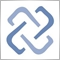7
14 755

### Introduction

Let's consider a  task of improving an indicator, which is applied to values of another indicator. In this article we'll continue to work with True Strength Index (TSI), which was created and considered in the previous article "MQL5: Create Your Own Indicator".

### Custom Indicator Based on Other Indicator's Values

When writing an indicator that uses the short form of the OnCalculate() function call, you might miss the fact that an indicator can be calculated not only by price data, but also by data of some other indicator (no matter whether it is a built-in or custom one).

Let's make a simple experiment: attach the built-in RSI indicator with standard settings to a chart, and drag the True_Strength_Index_ver2.mq5 custom indicator to the RSI indicator window. In the Parameters tab of the appeared window specify that the indicator should be applied to Previous Indicator's Data (RSI(14)).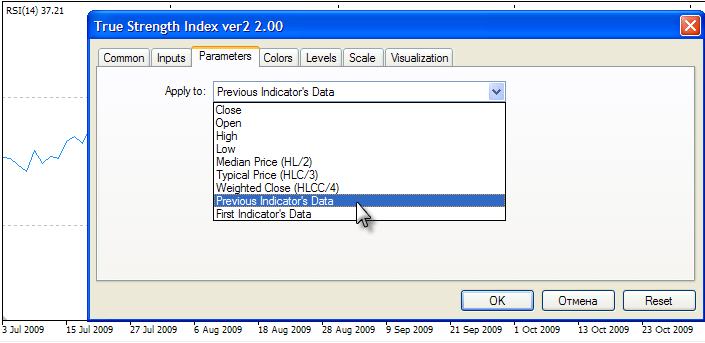The result will pretty much differ from what we've expected. The additional line of TSI indicator didn't appear in RSI indicator window, and in Data Window you'll find that its values are also unclear.

Despite the fact that values of RSI are defined almost throughout the whole history, the values of TSI (applied to RSI data) are either completely absent (in the beginning) or always equal to -100: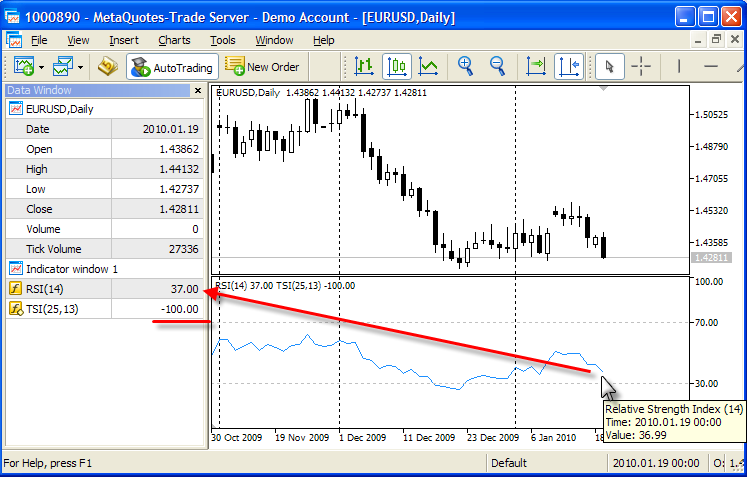Such a behavior is caused by the fact that the value of begin parameter is not used anywhere in OnCalculate() of our True_Strength_Index_ver2.mq5. The begin  parameter specifies the number of empty values in price[] input parameter. These empty values can't be used in the calculation of indicator values. Let's remind the definition of the first form of the OnCalculate() function call.
```int OnCalculate (const int rates_total,      // price[] array length
const int prev_calculated,  // number of bars calculated after previous call
const int begin,            // start index of meaningful data
const double& price[]       // array for calculation
);```

When applying the indicator to the price data specifying one of price constants, begin parameter is equal to 0, because there is a specified price type for each bar. Therefore the price[]  input array always has correct data beginning from its first element price. But if we specify data of another indicator as a source for calculations, it's no longer guaranteed.

### The begin Parameter of OnCalculate()

Let's check the values contained in the price[] array, if calculation is carried out using data of another indicator. To do so, in OnCalculate() function we will add some code, that will output the values we want to check. Now the beginning of OnCalculate() function looks like:

```//+------------------------------------------------------------------+
//| Custom indicator iteration function                              |
//+------------------------------------------------------------------+
int OnCalculate (const int rates_total,    // price[] array length;
const int prev_calculated,// number of available bars after previous call;
const int begin,          // start index of meaningful data in price[] array
const double &price[])    // data array, that will be used for calculations;
{
//--- flag for single output of price[] values
static bool printed=false;
//--- if begin isn't zero, then there are some values that we shouldn't take into account

if(begin>0 && !printed)
{
//--- let's output them
Print("Data for calculation begin from index equal to ",begin,
"   price[] array length =",rates_total);

//--- let's show the values that we shouldn't take into account for calculation
for(int i=0;i<=begin;i++)
{
Print("i =",i,"  value =",price[i]);
}
//--- set printed flag to confirm that we have already logged the values
printed=true;
}```

Once again let's drag'n'drop the modified version of our indicator to RSI(14) window and specify data of the previous indicator for calculation. Now we will see the values that aren't plotted and shouldn't be taken into account for calculations, where the values of the RSI(14) indicator are used.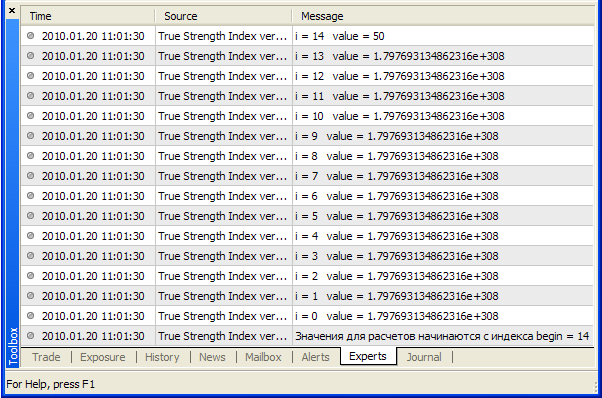### Empty Values in Indicator Buffers and DBL_MAX

The first 14 elements of the price[] array with indexes from 0 to 13 inclusive have the same value equal to 1.797693134862316e+308. You will encounter this number very often, because it is numerical value of built-in EMPTY_VALUE constant, that is used to point out empty values in an indicator buffer.

Filling empty values with zeros isn't a universal solution, because this value can be the result of calculation by some other indicators. For this reason all built-in indicators of the client terminal return this number for empty values. The value 1.797693134862316e+308 has been chosen because it is maximum possible value of double type, and for the convenience it's presented as DBL_MAX constant in MQL5.

To check whether a certain double type number is empty or not, you can compare it with the EMPTY_VALUE or constants. Both variants are equal, but it's better to use EMPTY_VALUE constant in your code to make it clear.

```//+------------------------------------------------------------------+
//| returns true for "empty" values                               |
//+------------------------------------------------------------------+
bool isEmptyValue(double value_to_check)
{
//--- if the value is equal DBL_MAX, it has an empty value
if(value_to_check==EMPTY_VALUE) return(true);
//--- it isn't equal DBL_MAX
return(false);
}```

The DBL_MAX is a very huge number and RSI indicator inherently can't return such values! And only the fifteenth element of the array (with index 14) has a reasonable value equal to 50. So, even if we don't know anything about the indicator as a source of data to be calculated, using begin parameter we can organize the data processing properly in such cases. To be more precise, we must avoid using these empty values in our calculations.

### Relationship between begin Parameter and PLOT_DRAW_BEGIN Property

It should be noted, that there is a close relationship between begin parameter, that is transfered to OnCalculate() function, and PLOT_DRAW_BEGIN property, that defines the number of initial bars without drawing. If we look into the source code of RSI from MetaTrader5 standard package, we'll see the following code in OnInit() function:
```//--- sets first bar from what index will be drawn
PlotIndexSetInteger(0,PLOT_DRAW_BEGIN,ExtPeriodRSI);```

This means that graphical plotting with index 0 begins only from the bar that has index equal to ExtPeriodRSI (it's an input variable, that specifies the period of RSI indicator), and there is no plotting of earlier bars.

In MQL5 language the calculation of some indicator A based on the data of indicator B is always performed on zero buffer values of indicator B. Zero buffer values of indicator B are transfered as a price[] input parameter to OnCalculate() function of indicator A. Inherently, zero buffer is assigned to zero graphical plotting with SetIndexBuffer() function. Therefore:

Rule of transferring PLOT_DRAW_BEGIN property to begin parameter: For calculations of custom indicator A based on the data of other (base) indicator B, the value of begin input parameter in OnCalculate() function is always equal to the value of PLOT_DRAW_BEGIN property of base indicator's B zero graphical plotting.

So, if we have created RSI indicator (indicator B) with period 14 and then created our custom indicator True Strength Index (Indicator A) based on it's data, then:

• RSI (14) indicator is plotted starting from 14th bar because of PlotIndexSetInteger(0,PLOT_DRAW_BEGIN,14);
• price[] input array in OnCalculate() function contains values of RSI indicator's zero buffer;
• value of begin input parameter in OnCalculate() function of TSI indicator is obtained from PLOT_DRAW_BEGIN property of RSI indicator's zero graphical plotting.

Remember that TSI indicator is not drawn from the beginning of chart, because the indicator value is not determined for some first bars. The first bar index, that will be plotted as line in TSI indicator, is equal to r+s-1, where:

• r - period of first exponential smoothing of MTMBuffer[] and AbsMTMBuffer[] arrays into corresponding EMA_MTMBuffer[] and EMA_AbsMTMBuffer[] arrays;
• s - period of the subsequent smoothing of EMA_MTMBuffer[] and EMA_AbsMTMBuffer[] arrays.

For bars with indexes less than r+s-1 there are no values to plot TSI indicator. Therefore, for final MA2_MTMBuffer[] and EMA2_AbsMTMBuffer[] arrays used to calculate TSI indicator, the data have additional offset and begin from r+s-1 index. You can find more information in "MQL5: Create Your Own Indicator" article.

There is a statement in OnInit() function to disable drawing of first r+s-1 bars:

```//--- first bar to draw
PlotIndexSetInteger(0,PLOT_DRAW_BEGIN,r+s-1);```

As the beginning of input data has shifted forward by begin bars, we must take it into consideration and increase beginning position of data drawing by begin bars in OnCalculate() function:

```   if(prev_calculated==0)
{
//--- let's increase beginning position of data by begin bars,
//--- because we use other indicator's data for calculation
if(begin>0)PlotIndexSetInteger(0,PLOT_DRAW_BEGIN,begin+r+s-1);
}```
Now we are taking into consideration begin parameter to calculate the values of TSI indicator. Moreover, begin parameter will be transfered properly if some other indicator will use values of TSI for calculation: beginother_indicator=beginour_indicator+r+s-1. Therefore, we can formulate the rule of imposing one indicator on values of other indicator:

Rule of indicators imposing : If some custom indicator A is drawn starting from Na position (first Na values are not plotted) and is based on data of other indicator B, that is drawn from position Nb, the resulting indicator A{B} will be drawn starting from Nab=Na+Nb position, where A{B} means that indicator A is calculated on zero buffer values of indicator B.

Therefore, TSI (25,13) {RSI (14)} means that TSI (25,13) indicator is made of values of the RSI (14) indicator. As a result of imposing, now the beginning of data is (25+13-1)+14=51. In other words, indicator's drawing will begin from the 52th bar (the bars indexation begins with 0).

### Adding begin Values to Use in Indicator Calculations

Now we know exactly, that meaningful values of price[] array always start form position, specified by begin parameter. Let's modify our code step by step. First comes the code that calculates MTMBuffer[] and AbsMTMBuffer[] arrays values. Without begin parameter array filling started with index 1.

```//--- calculate values for mtm and |mtm|
int start;
if(prev_calculated==0) start=1;  // start filling MTMBuffer[] and AbsMTMBuffer[] arrays from 1st index
else start=prev_calculated-1;    // set start equal to last array index
for(int i=start;i<rates_total;i++)
{
MTMBuffer[i]=price[i]-price[i-1];
AbsMTMBuffer[i]=fabs(MTMBuffer[i]);
}```

Now we'll start from (begin+1) position, and modified code looks like the following (code changes are outlined with bold):

```//--- calculate values for mtm and |mtm|
int start;
if(prev_calculated==0) start=begin+1;  // start filling MTMBuffer[] and AbsMTMBuffer[] arrays from begin+1 index
else start=prev_calculated-1;           // set start equal to the last array index
for(int i=start;i<rates_total;i++)
{
MTMBuffer[i]=price[i]-price[i-1];
AbsMTMBuffer[i]=fabs(MTMBuffer[i]);
}
```

As values from price to price[begin-1] can't be used for calculations, we begin from price[begin]. The first values calculated for MTMBuffer[] and AbsMTMBuffer[] arrays will look like following:

```      MTMBuffer[begin+1]=price[begin+1]-price[begin];
AbsMTMBuffer[begin+1]=fabs(MTMBuffer[begin+1]);```

For this reason, start variable in for cycle now has initial value start=begin+1, instead of 1.

### Account for begin for Dependent Arrays

Now comes exponential smoothing of MTMBuffer[] and AbsMTMBuffer[] arrays. The rule is also simple: if the initial position of base array has increased by begin bars, then the initial position for all dependent arrays should be increased by begin bars also.

```//--- calculating the first moving average on arrays
ExponentialMAOnBuffer(rates_total,prev_calculated,
1,               // index of the starting element in array
r,               // period of exponential average
MTMBuffer,       // source buffer for average
EMA_MTMBuffer);  // target buffer
ExponentialMAOnBuffer(rates_total,prev_calculated,
1,r,AbsMTMBuffer,EMA_AbsMTMBuffer);```

Here, MTMBuffer [] and AbsMTMBuffer [] are basis arrays, and calculated values in these arrays now start from index that is greater by begin. So, we'll just add this offset to ExponentialMAOnBuffer() function.

Now, this block looks like this:

```//--- calculating the first moving average on arrays
ExponentialMAOnBuffer(rates_total,prev_calculated,
begin+1,        // index of the starting element in array
r,               // period for exponential average
MTMBuffer,       // source buffer for average
EMA_MTMBuffer);  // target buffer
ExponentialMAOnBuffer(rates_total,prev_calculated,
begin+1,r,AbsMTMBuffer,EMA_AbsMTMBuffer);
```

As you can see, the whole modification was to accommodate the increase of start data position, defined by begin parameter. Nothing complicated. In the same way we'll change the second block of smoothing.

Before:

```//--- calculating the second moving average on arrays
ExponentialMAOnBuffer(rates_total,prev_calculated,
r,s,EMA_MTMBuffer,EMA2_MTMBuffer);
ExponentialMAOnBuffer(rates_total,prev_calculated,
r,s,EMA_AbsMTMBuffer,EMA2_AbsMTMBuffer);```

After:

```//--- calculating the second moving average on arrays
ExponentialMAOnBuffer(rates_total,prev_calculated,
begin+r,s,EMA_MTMBuffer,EMA2_MTMBuffer);
ExponentialMAOnBuffer(rates_total,prev_calculated,
begin+r,s,EMA_AbsMTMBuffer,EMA2_AbsMTMBuffer);```

In the same way we'll change the last calculation block.

Before:

```//--- calculating values of our indicator
if(prev_calculated==0) start=r+s-1; // set initial index for input arrays
else start=prev_calculated-1;       // set start equal to last array index
for(int i=start;i<rates_total;i++)
{
TSIBuffer[i]=100*EMA2_MTMBuffer[i]/EMA2_AbsMTMBuffer[i];
}```

After:

```//--- calculating values of our indicator
if(prev_calculated==0) start=begin+r+s-1; // set initial index for input arrays
else start=prev_calculated-1;              // set start equal to last array index
for(int i=start;i<rates_total;i++)
{
TSIBuffer[i]=100*EMA2_MTMBuffer[i]/EMA2_AbsMTMBuffer[i];
}
```

Tuning of the indicator is over, and now it will skip the first begin empty values of price[] input array in the OnCalculate() and will take into account the offset caused by this omission. But we have to remember that some other indicators can use TSI values for calculations. For this reason, we will set the empty values of our indicator to EMPTY_VALUE.

### Is the Initialization of Indicator Buffers Necessary?

In MQL5 arrays are not initialized by default with some defined values. The same is applied to arrays, that are specified by SetIndexBuffer() function to indicator buffers. If an array is an indicator buffer, its size will depend on the value of the rates_total parameter in the OnCalculate() function;

You might be tempted to initialize all indicator buffers with EMPTY_VALUE values using ArrayInitialize() function, for example, at once in the beginning of OnCalculate():

```//--- if it is the first call of OnCalculate()
if(prev_calculated==0)
{
ArrayInitialize(TSIBuffer,EMPTY_VALUE);
}
```

But it is not recommended because of the following reason: While client terminal is working, new quotes are received for the symbol, whose data are used to calculate the indicator. After some time the number of bars will increase, so the client terminal will reserve additional memory for the indicator buffers.

But the values of new ("attached") array elements may have any value, since during memory reallocation for any array the initialization is not carried out. Initial initialization can give you a false sure, that all array elements that have not been explicitly defined, will be filled with values that have been specified during initialization. Of course it's not true, and you should never think that numerical value of variable or some array element will be initialized with value necessary to us.

You should set the value for each element of the indicator buffer. If the values of some bars aren't defined by the indicator algorithm, you should set them explicitly with empty value. For example, if some value of the indicator buffer is calculated by the divide operation, in some cases the divisor can be zero.

We know, that division by zero is a critical runtime error in MQL5 and it leads to an immediate termination of a mql5-program. Instead of avoiding division by zero by handling this special case in code, it's necessary to set the value for this buffer element. Maybe, it is better to use value that we have assigned as empty for this drawing style.

For example, for some drawing style we have defined zero as empty value using PlotIndexSetDouble() function:

```   PlotIndexSetDouble(plotting_style_index,PLOT_EMPTY_VALUE,0);
```

Then for all empty values of the indicator buffer in this drawing it's necessary to define zero value explicitly:

```   if(divider==0)
IndicatorBuffer[i]=0;
else
IndicatorBuffer[i]=...
```

Besides, if DRAW_BEGIN has been specified for some drawing, all elements of the indicator buffer with indexes from 0 to DRAW_BEGIN will be filled with zeros automatically.

### Conclusion

So, let's make a brief summary. There are some necessary conditions for indicator to be correctly calculated based on data of another indicator (and to make it suitable for use in other mql5-programs):

1. Empty values in built-in indicators are filled with the values of EMPTY_VALUE constant, that is exactly equal to the maximum value for double type (DBL_MAX).
2. For details about the start index of meaningful values of an indicator you should analyze the begin input parameter of the short form of OnCalculate().
3. In order to prohibit the drawing of first N values for the drawing style specified, set DRAW_BEGIN parameter using the following code:
`PlotIndexSetInteger(plotting_style_index,PLOT_DRAW_BEGIN,N);`
4. If DRAW_BEGIN is specified for some drawing, all of the indicator buffer elements with indexes from 0 to DRAW_BEGIN will be filled automatically with empty values (default is EMPTY_VALUE).
5. In OnCalculate() function add an additional offset by begin bars for correct use of other indicator data in your own indicator:
```//--- if it's the first call
if(prev_calculated==0)
{
//--- increase position of data beginning by begin bars,
//--- because of other indicator's data use
if(begin>0)PlotIndexSetInteger(plotting_style_index,PLOT_DRAW_BEGIN,begin+N);
}
```
6. You can specify your own empty value that differs from EMPTY_VALUE in OnInit() function using the following code:
`PlotIndexSetDouble(plotting_style_index,PLOT_EMPTY_VALUE,your_empty_value);`
7. Don't rely on a one-time initialization of the indicator buffers using the following code:
```ArrayInitialize(buffer_number,value);
```
You should set all values of the indicator buffer for OnCalculate() function explicitly and consistently, including empty values .

Of course, in future, when you have some experience in writing indicators, you will encounter some cases that are beyond the scope of this article, but I hope at that time your knowledge of MQL5 will allow you to solve them.

Translated from Russian by MetaQuotes Software Corp.

Translated from Russian by MetaQuotes Software Corp.
Original article: https://www.mql5.com/ru/articles/15

Attached files |

#### Other articles by this author

Last comments | Go to discussion (7)| 18 Dec 2010 at 17:51

Hi,

I've understood the problem: you are only able to apply one indicator to another which is in the same window.

Do you have an idea on how to apply one indicator (and to display it in a separate window) to another which is in the main window?

thanks

Bests| 7 Jan 2012 at 15:54

Hi,

This is a very good article but I have some questions.

1.  Only the indicators that uses the short form of the OnCalculate() function call, can be applaied to another indicator or the indicators that use the long form of the OnCalculate() function call can be applied too? If so , haw can be applied one indicator with long form of the OnCalculate() function call, to another indicator???

2. Applying one indicator to another (TSI over RSI) generates some display problems (see attached image). While RSI is plotted from 0 to 100 the second indicator (TSI) have values below 0 too , values wich are not visibile on the chart. Wich is the problem and why the chart don't properly adjust to display correctly both indicators. Can we solve this problem or it's a MetaTrader bug???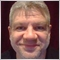| 4 Jul 2013 at 17:02
paul.necsoiu:

Hi,

This is a very good article but I have some questions.

1.  Only the indicators that uses the short form of the OnCalculate() function call, can be applaied to another indicator or the indicators that use the long form of the OnCalculate() function call can be applied too? If so , haw can be applied one indicator with long form of the OnCalculate() function call, to another indicator???

2. Applying one indicator to another (TSI over RSI) generates some display problems (see attached image). While RSI is plotted from 0 to 100 the second indicator (TSI) have values below 0 too , values wich are not visibile on the chart. Wich is the problem and why the chart don't properly adjust to display correctly both indicators. Can we solve this problem or it's a MetaTrader bug???

1. Yes only indicator that uses the short form of OnCalculate can be applied to another indicator data.

2. You have to edit to properties of RSI indicator and change "Fixed minimum" on the Scale tab to -50 instead of 0.| 13 Jul 2015 at 19:22
I am somewhat a newbie in mql programming...my question is how do i make an indicator that checks the upper bollinger band value at a specific bar,whether at that point the bollinger band value is between the open and close of that bar after which it plots a down arrow above the current bar.. if close>upperbollingvalue>open .. I have tried searchng around but no luck..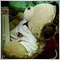| 15 Nov 2020 at 15:50

Hi,

Using a simple chart, I can drag Indicator A on a sub window.  Indicator B can be dragged into Indicator A and "Apply To" can be "Previous Indicator Data".

Both Indicator A and B are Custom Indicators.

When trying to Code EA, if using a standard MA for indicator B, you can pass the indicator handle in Applied Price.  However, for Custom Indicators, the method is to pass it as the last parameter for the creation of Indicator B.

1. What are the things to look out for in Indicator B to know if it will accept the assignment of an indicator handle as the data series to process on?

2. In the EA, if Indicator B continues to open in main window and attaches to Price Close, what can i check?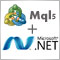How to Export Quotes from МetaTrader 5 to .NET Applications Using WCF Services
Want to organize export of quotes from MetaTrader 5 to your own application? The MQL5-DLL junction allows to create such solutions! This article will show you one of the ways to export quotes from MetaTrader 5 to applications written in .NET. For me it was more interesting, rational and easy to implement export of quotes using this very platform. Unfortunately, version 5 still does not support .NET, so like in old days we will use win32 dll with .NET support as an interlayer.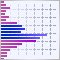The Price Histogram (Market Profile) and its implementation in MQL5
The Market Profile was developed by trully brilliant thinker Peter Steidlmayer. He suggested to use the alternative representation of information about "horizontal" and "vertical" market movements that leads to completely different set of models. He assumed that there is an underlying pulse of the market or a fundamental pattern called the cycle of equilibrium and disequilibrium. In this article I will consider Price Histogram — a simplified model of Market Profile, and will describe its implementation in MQL5.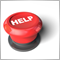MQL5.community - User Memo
You have just registered and most likely you have questions such as, "How do I insert a picture to my a message?" "How do I format my MQL5 source code?" "Where are my personal messages kept?" You may have many other questions. In this article, we have prepared some hands-on tips that will help you get accustomed in MQL5.community and take full advantage of its available features.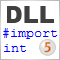How to Exchange Data: A DLL for MQL5 in 10 Minutes
Now not so many developers remember how to write a simple DLL, and what are special features of different system binding. Using several examples, I will try to show the entire process of the simple DLL's creation in 10 minutes, as well as to discuss some technical details of our binding implementation. I will show the step-by-step process of DLL creation in Visual Studio with examples of exchanging different types of variables (numbers, arrays, strings, etc.). Besides I will explain how to protect your client terminal from crashes in custom DLLs.